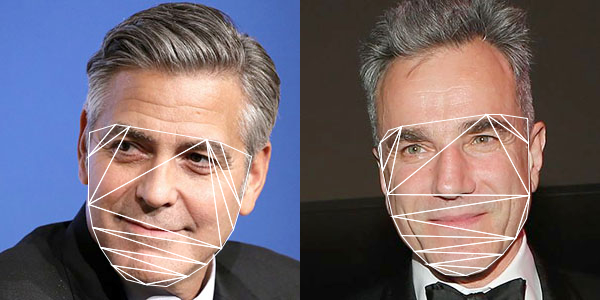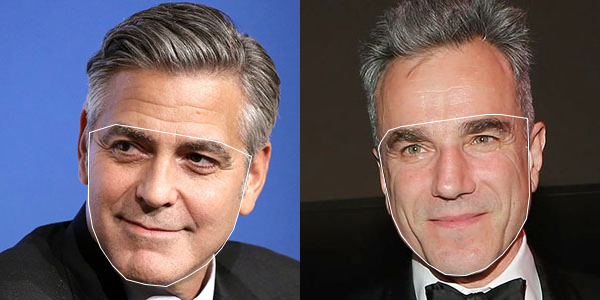## Face swap example in OpenCV with Processing (v.2)

To enhance the last post in face swap, we can make use of the cloning features of the Photo module in OpenCV. The command we use is the seamlessClone() function.

`Photo.seamlessClone(warp, im2, mask, centre, output, Photo.NORMAL_CLONE);`

where warp is the accumulation of all warped triangles; im2 is the original target image; mask is the masked image of the convex hull of the face contour; centre is a Point variable of the centre of the target image; output will be the blended final image.Complete source code is now in the GitHub repository, ml20180820b.

## Face swap example in OpenCV with Processing (v.1)

After the previous 4 exercises, we can start to work on with the OpenCV face swap example in Processing. With the two images, we first compute the face landmark for each of them. We then prepare the Delaunay triangulation for the 2nd image.  Based on the triangles in the 2nd image, we find corresponding vertices in the 1st image. For each triangle pair, we perform the warp affine transform from the 1st image to the 2nd image. It will create the face swap effect.Note the skin tone discrepancy in the 3rd image for the face swap.

Full source code is now available at the GitHub repository ml20180820a.

## Delaunay triangulation of the face contour in OpenCV with Processing

The 4th exercise is a demonstration of the planar subdivision function in OpenCV to retrieve the Delaunay triangulation of the face convex hull outline that we obtain from the last post. The program will use the Subdiv2D class from the Imgproc module in OpenCV.

`Subdiv2D subdiv = new Subdiv2D(r);`

where r is am OpenCV Rect object instance defining the size of the region. It is usually the size of the image we are working on. For every point on the convex hull, we add it to the subdiv object by,

`subdiv.insert(pt);`

where pt is an OpenCV Point object instance. To obtain the Delaunay triangles, we use the following codes,

```MatOfFloat6 triangleList = new MatOfFloat6(); subdiv.getTriangleList(triangleList); float [] triangleArray = triangleList.toArray();```

The function getTriangleList() will compute the Delaunay triangulation based on all the points inserted. It will return the result in the variable, triangleList. This variable is an instance of MatOfFloat6, and which is a collection of 6 numbers. The first pair of numbers are the x and y position of the first vertex of the triangle. The second pair of numbers are for the second vertex. The third pair of numbers are for the third vertex of the triangle. Based on this, we can draw each triangle in the Delaunay triangulation process, as shown in the image below.Complete source code is now available in my GitHub repository at ml20180819b.

## Face landmark convex hull detection in OpenCV with Processing

The 3rd exercise is the demonstration of obtaining the convex hull of the face landmark points in the OpenCV Face module. The program based on the face landmark information collected from the last post to find out the convex hull of the face detected.

The function is provided by the Imgproc (image processing) module of OpenCV. In the sample program, the following command will obtain the each point information of those points on the convex hull of the polygon.

`Imgproc.convexHull(new MatOfPoint(p), index, false);`

The first parameter, variable p is an array of type Point in OpenCV. The second parameter, index, is the returned value of type MatOfInt indicating all the points along the convex hull boundary. The integer value is the index in the original array p. The third parameter, false, indicates the clockwise orientation is false. By traversing the array index, we can obtain all the points along the convex hull.The complete source code is now in my GitHub repository ml20180819a.